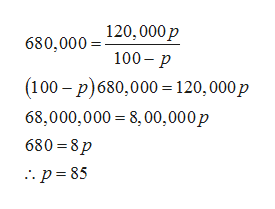# The cost C in dollars of removing p% of the air pollutants in the stack emissions of a utility company is modeled by the equation below. Determine the percent of air pollutants  in the stack emissions that can be removed for \$680,000.C= 120,000p / 100-p

Question

The cost C in dollars of removing p% of the air pollutants in the stack emissions of a utility company is modeled by the equation below. Determine the percent of air pollutants  in the stack emissions that can be removed for \$680,000.

C= 120,000p / 100-p

check_circle

Step 1

The given equation of the cost C (in dollars) in removing p percent of the air pollutants in the stack emissions is,

Step 2

When the cost is 680,000 dollars, then the percentage of the ...help_outlineImage Transcriptionclose120,000p 680,000= 100 p (100 p)680,000 = 120,000p 68,000,000 = 8,00,00 0p 680=8p p 85 fullscreen

### Want to see the full answer?

See Solution

#### Want to see this answer and more?

Solutions are written by subject experts who are available 24/7. Questions are typically answered within 1 hour.*

See Solution
*Response times may vary by subject and question.
Tagged in

### Equations and In-equations